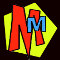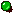# PRIME NUMBERS

## Prime numbers are numbers that can only be divided by themselves or by 1.

#### 13 is a prime number because it will only divide exactly by 1 or 13. If you have 13 eggs, the only box they will neatly fit into has one row of 13 eggs. If you try boxes that are shorter and wider, you'll never neatly fit all 13 eggs in.

##### The prime numbers smaller than 100 are: 2, 3, 5, 7, 11, 13, 17, 19, 23, 29, 31, 37, 41, 43, 47, 53, 59, 61, 67, 71, 73, 79, 83, 89, 97 ... ...and they go on for ever. So far nobody has found a pattern to predict them and they can't decide if 1 is prime or not!Mathematicians LOVE prime numbers because just about all of maths is made out of prime numbers. In the same way, scientists LOVE atoms because just about everything we know is made out of atoms.

You can find out how the ancient Greeks found prime numbers up to 100 and beyond using the Sieve of Eratosthenes.

So now we know what prime numbers are, what can we do with them?

• Smashing big sums to bits! If you've read The Mean and Vulgar Bits then you'll know how to use prime numbers to make ugly divisions and fractions a lot easier.
• Secret Codes! Some of the toughest codes in the world are made using mega prime numbers. You can find how this works in The Secret Life of Codes. There's even a VERY simple prime code in the original Murderous Maths book.
• A good trick....

Before we see the trick, here's a neat toy for you to try. It's a special calculator which can tell you if a number is prime or not. All you need to do is type a number in the box, and then hit the ACTIVATE button. (Warning: if you put in numbers with lots and lots of digits your computer might get a bit cross!)

##### The Prime Tester was invented by Abraham Joffe
 THE PRIME TESTER Thinking... [0%] Thinking... [0%] Type a whole number into the box.
Have you tried it out?

What's the BIGGEST prime number you can find?

 If you want to see the BIGGEST prime number we know about, check theGREAT INTERNET MERSENNE PRIME SEARCH## The Prime Number Trick

Using prime numbers, you can amaze your friends with a prime prediction...

• Ask your friends to pick any prime number bigger than 5, but they must not tell you what it is.
• Square it. (In other words multiply the prime number by itself.)
• Divide by 12

Without knowing which prime number your friends picked, you can still tell them:

There will be a remainder of 6.

(Or if they do the sum on a calculator, the answer will end with " .5")

If you want to try this out yourself right now, use the Prime Number Calculator to find a nice big juicy prime number.
Put your number into this normal calculator (you need to push the buttons with your mouse). First you multiply your prime number by itself then add 17 and finally divide by 12. What do you get?